# DAV Class 8 Maths Chapter 2 Worksheet 1 Solutions

The DAV Class 8 Maths Book Solutions and DAV Class 8 Maths Chapter 2 Worksheet 1 Solutions of Cubes and Cube Roots offer comprehensive answers to textbook questions.

## DAV Class 8 Maths Ch 2 WS 1 Solutions

Question 1.
Find the cubes of:
(i) 8
(ii) 13
(iii) 17
(iv) 1.3
(v) 0.06
(vi) 0.4
(vii) $$\frac{2}{3}$$
(viii) – 7
(ix) – 9
(x) – 12
Solution:
(i) 83 = 8 × 8 × 8 = 512
(ii) 133 = 13 × 13 × 13 = 2197
(iii) 173 = 17 × 17 × 17 = 4913
(iv) 1.33 = 1.3 × 1.3 × 1.3 = 2.197
(v) 0.063 = 0.000216
(vi) (0.4)2 = 0.064
(vii) $$\left(\frac{2}{3}\right)^3=\frac{2 \times 2 \times 2}{3 \times 3 \times 3}=\frac{8}{27}$$
(viii) (- 7)3 = (- 7) × (- 7) × (- 7) = – 343
(ix) (- 9)3 = (- 9) × (- 9) × (- 9) = – 729
(x) (- 12)3 = (- 12) × (- 12) × (- 12) = – 1728.Question 2.
Which of the following numbers are perfect cubes?
(i) 4096
(ii) 108
(iii) 392
(iv) – 27000
(v) $$\frac{-64}{1331}$$
Solution:
(i) 4096∴ 4096 = 2 × 2 × 2 × 2 × 2 × 2 × 2 × 2 × 2 × 2 × 2 × 2
= 23 × 23 × 23
∴ 4096 is a perfect cube.

(ii) 108108 = 2 × 2 × 3 × 3 × 3
= 2 × 2 × 33
Here 2 × 2 is left without triplet.
∴ 108 is not a perfect cube.(iii) 392392 = 2 × 2 × 2 × 7 × 7
= 23 × 7 × 7
Here 7 × 7 is left without triplet.
∴ 392 is not a perfect cube.

(iv) – 27000 = (- 2) × (- 2) × (- 2) × (- 3) × (- 3) × (- 3) × (- 5) × (- 5) × (- 5)
= (- 2) × (- 3)3 × (- 5)Here all have triplets.
∴ – 27000 is a perfect cube.

(v) $$\frac{-64}{1331}$$
⇒ – 64 = (- 4) × (- 4) × (- 4)
and 1331 = 11 × 11 × 11
∴ $$\frac{-64}{1331}$$ is a perfect square.Question 3.
Find the smallest number by which 2560 must be multiplied so that the product is a perfect cube.
Solution:
∴ 2560 = 2 × 2 × 2 × 2 × 2 × 2 × 2 × 2 × 2 × 5Here 5 is left out without triplet.
So for this triplet 5 × 5 is needed
∴ 5 × 5 = 25 is the smallest number by which the given 2560 number must be multiplied to make it a perfect cube.

Question 4.
Find the smallest number by which 8788 be divided so that the quotient is a perfect cube.
Solution:
∴ 8788 = 2 × 2 × 13 × 13 × 13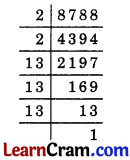Here 2 × 2 is left out for making a triplet
∴ 2 × 2 = 4 is the smallest number by which the given number must be divided to make it a perfect cube.Question 5.
Write True or False for the following statements.

(i) 650 is not a perfect cube.
Solution:
True

(ii) Perfect cubes may end with two zeros.
Solution:
False

(iii) Perfect cubes of odd numbers may not always be odd.
Solution:
False

(iv) Cube of negative numbers are negative.
Solution:
True

(v) For a number to be a perfect cube, it must have prime factors in triplets.
Solution:
FalseQuestion 1.
Using the following matter, fill in the blanks:
(i) 1 = 1 = 13
(ii) 3 + 5 = 8 = 22
(iii) 7 + 19 + 11 = ____ = ____
(iv) 13 + 15 + 17 + 19 = ____ = ____
(v) ____ = 125 = 53
Sol.
(iii) 7 + 9 + 11 = 27 = 33
(iv) 13 + 15 + 17 + 19 = 64 = 43
(u) 21 + 23 + 25 + 27 + 29 = 125 = 53

Question 2.
Using the given matter, fill in the blanks:
(i) 23 – 13 = 1 + 2 × 1 × 3 = 7
(ii) 33 – 23 = 1 + 3 × 2 × 3 = 19
(iii) 43 – 33 = ____ = ____
(iv) 53 – 43 = ____ = ____
(v) 123 – 113 = ____ = ____
(vi) 203 – 193 = ____ = ____
Solution:
(iii) 43 – 33 = 1 + 4 × 3 × 3 = 37
(iv) 53 – 43 = 1 + 5 × 4 × 3 = 61
(v) 123 – 113 = 1 + 12 × 11 × 3 = 397
(vi) 203 – 193 = 1 + 20 × 19 × 3 = 1141Question 3.
Which of the following are perfect cubes? Also find their cube roots.
(i) 6859
(ii) 2025
(iii) 15625
(iv) 3375
Solution:
(i) ∴ 6859 = 19 × 19 × 19
= $$\sqrt{19^3}$$ = 19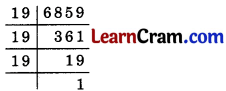Here 6859 is perfect cube and its cube root is 19.

(ii) 2025 = 3 × 3 × 3 × 3 × 5 × 5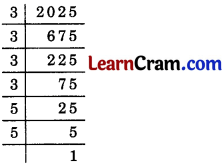Here 3 and 5 are ungrouped of triplet.
Hence, 2025 is not a perfect cube.

(iii) 15625 = 5 × 5 × 5 × 5 × 5 × 5
= 53 × 53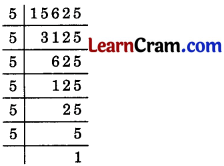∴ 15625 is a perfect cube.
Hence, its cube root is 5 × 5 = 25

(iv) 3375 = 3 × 3 × 3 × 5 × 5
= 33 × 53∴ 3375 is a perfect cube.
Hence, its cube root is 3 × 5 = 15.Question 4.
Find the cube root of 17576 by estimation method.
Solution:
The following steps are taken to proceed:
(i) Form groups of three, starting from the rightmost digit of 17576.
(ii) 17 576, here 576 has 3 digits whereas 17 has only two digits.
(iii) The digit 6 is at ones place. So we take the one’s place of the required cube root as 6.
(iv) Take the other group, i.e. 17. Cube of 2 is 8 and cube of 3 is 27
∴ 23 < 17 < 33
∴ The ten’s place is 2.
Hence the required cube root is 26.

Question 5.
Find the cube root of 13824 by prime factorisation method.
Solution: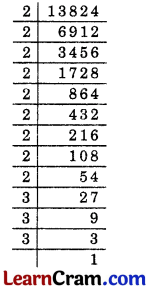13824 = 2 × 2 × 2 × 2 × 2 × 2 × 2 × 2 × 2 × 3 × 3 × 3
= 23 × 23 × 23 × 33
∴ $$\sqrt{13824}$$ = 2 × 2 × 2 × 3 = 24.### DAV Class 8 Maths Chapter 2 Worksheet 1 Notes

The cube of a number is obtained by multiplying itself three times and is read as, the number raised to the power 3.
e.g. cube of 4 = 43
= 4 × 4 × 4 = 64
cube of 7 = 73
= 7 × 7 × 7 = 343
cube of 9 = 93
= 9 × 9 × 9 = 729
Here 64, 343 and 729 are called perfect cubes.

Properties of Cubes of Numbers

1. The cube of any even number is even,
e.g. 43 = 64 (even),
83 = 512 (even)

2. The cube of any odd number is odd,
e.g. (3)3 = 27 (odd),
(9)3 = 729 (odd)

3. The cube of any number multiple of 2 is divisible by 8,
e.g. (6)3 = 216 (divisible by 8)
(8)3 = 512 (divisible by 8)
(12)3 = 1728 (divisible by 8)

4. The cube of a negative number is always negative,
e.g. (- 2) = – 2 × – 2 × – 2 = – 8
(- 5)3 = – 5 × – 5 × – 5 = – 125

5. The cube of a positive number is always positive,
e.g. (3)3= 27 (positive)
(4)3 = 64 (positive)

6. The cube of a rational number is equal to the cube of its numerator divided by the cube of its denominator,
e.g. $$\left(\frac{4}{5}\right)^3=\frac{4^3}{5^3}$$
= $$\frac{64}{125}$$

$$\left(\frac{3}{4}\right)^3=\frac{3^3}{4^3}$$
= $$\frac{27}{64}$$Example 1.
Is 512 a perfect cube? What is the number whose cube is 512?
Solution:
Resolving 512 into prime factors.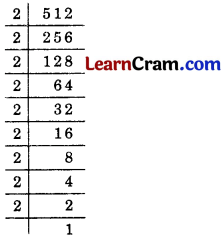∴ 512 = $$\underline{2 \times 2 \times 2} \times \underline{2 \times 2 \times 2} \times \underline{2 \times 2 \times 2}$$
512 = 23 × 23 × 23
512 = (2 × 2 × 2)3 = (8)
Hence, 512 is a perfect cube and 8 is the number whose cube is 512.

Example 2.
Is 1512 a perfect cube?
Solution:
Resolving 1512 into prime factors.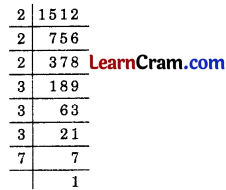1512 = $$2 \times 2 \times 2 \times 3 \times 3 \times 3$$ × 7
1512 = 23 × 23 × 7
Here 7 is ungrouped.
Hence, 1512 is not a perfect cube.Example 3.
Is 53240 a perfect cube? If not, then by which smallest natural number should 53240 be divided so that the quotient is a perfect cube.
Solution:
Resolving 53240 into prime factors.53240 = $$2 \times 2 \times 2 \times 5 \times \underline{11 \times 11 \times 11}$$
= 23 × 5 × 113
Here 5 is left ungrouped.
So 53240 is not a perfect cube.
∴ 53240 ÷ 5 = 10648
Hence, 10648 is a perfect cube.

Example 4.
Is 68600 a perfect cube? If not, find the smallest number by which 6860C must be multiplied to get a perfect cube.
Solution:
Let us resolve 68600 into prime factors.∴ 68600 = 2 × 2 × 2 × 5 × 5 × 7 × 7 × 7
= 23 × 5 × 5 × 7Here 5 × 5 is left without triplet.
∴ 68600 is not a perfect cube.
To make it a perfect cube, we multiply it by 5.
∴ 68600 × 5 = 2 × 2 × 2 × 5 × 5 × 5 × 7 × 7 × 7
= 343000 which is a perfect cube.
Hence, 5 is the number by which the given number must be multiplied 1 to make it a perfect cube.